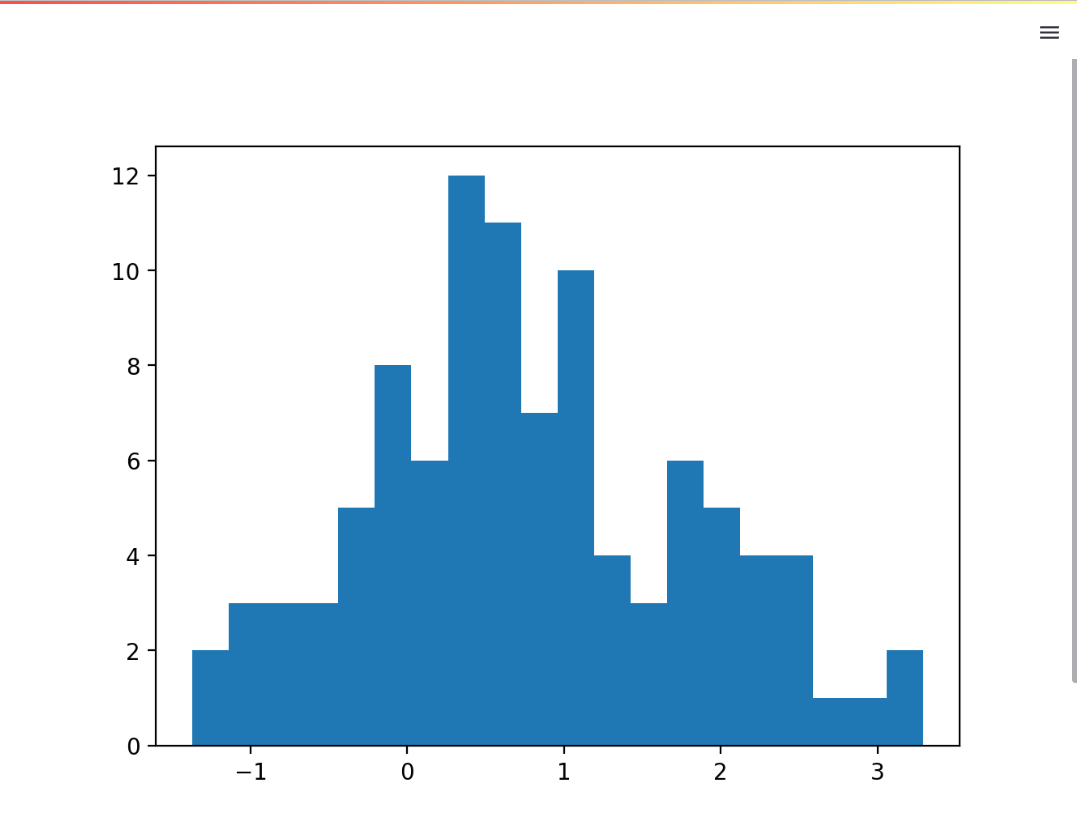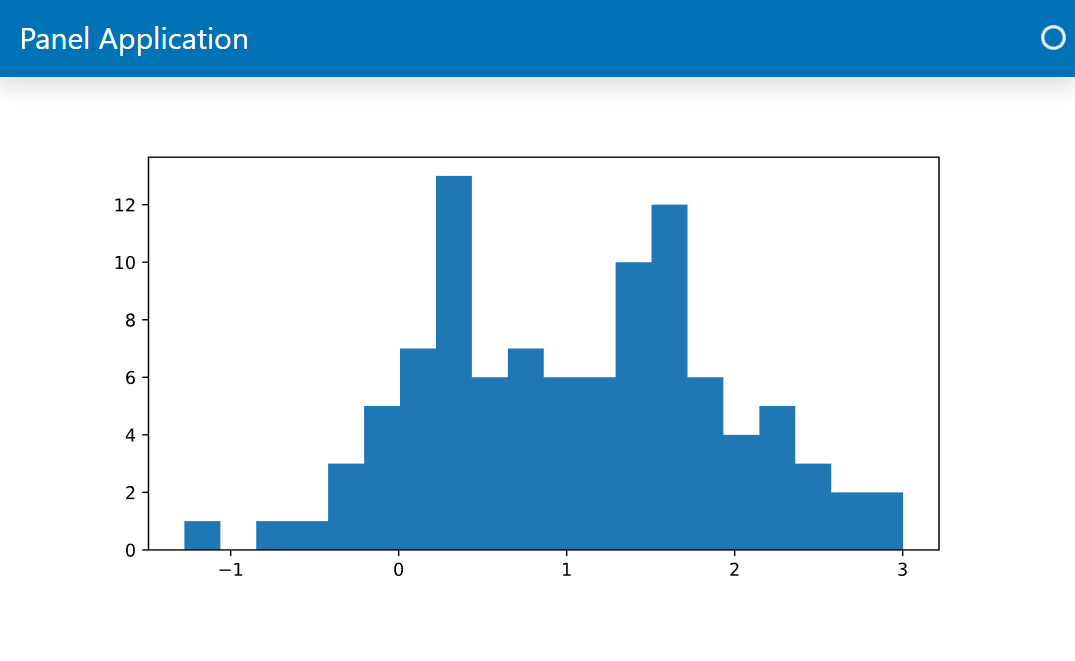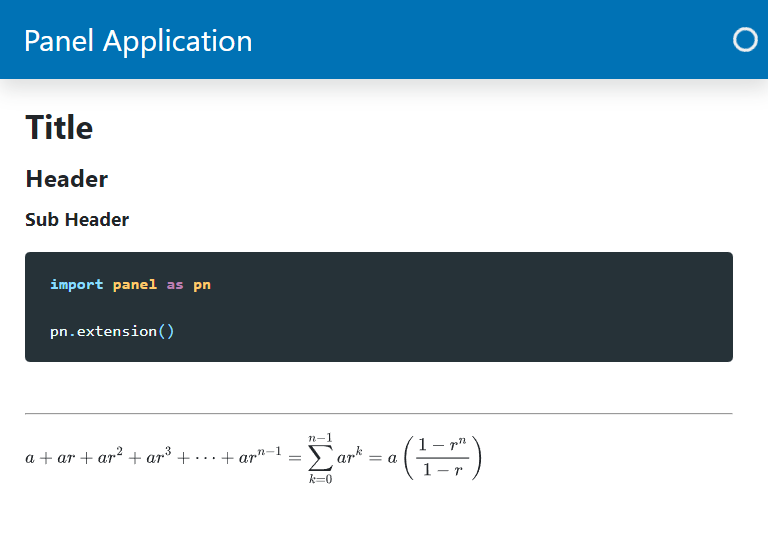# Displaying Content with Panes#

In Panel the objects that can display your Python objects are called panes. With Panels panes you will be able to:

• Get notifications about interactions like click events on your plots and tables and react to them.

• Use unique data visualization ecosystems like HoloViz, ipywidgets and VTK.

Check out the Panes Section of the Component Gallery for the full list of panes.

## Migration Steps#

To display content in Panel:

• Replace your Streamlit st.some_object snake cased functions with the corresponding Panel pn.pane.SomeObject CamelCased classes.

• Replace your Streamlit text elements with pn.pane.Markdown.

Identify the relevant Panel panes in the Panes Section of the Component Gallery.

## Examples#

### Matplotlib Pane#

#### Streamlit Matplotlib Example#

import numpy as np
import streamlit as st

import matplotlib.pyplot as plt

data = np.random.normal(1, 1, size=100)
fig, ax = plt.subplots(figsize=(8,4))
ax.hist(data, bins=20)

st.pyplot(fig)#### Panel Matplotlib Example#

You will find Panels panes in the pn.pane module.

We use Matplotlibs Figure interface instead of the pyplot interface to avoid memory leaks if you forget to close the figure. This is all described in the Matplotlib Guide.

import panel as pn
import numpy as np

from matplotlib.figure import Figure

pn.extension(sizing_mode="stretch_width", template="bootstrap")

data = np.random.normal(1, 1, size=100)
fig = Figure(figsize=(8,4))
ax = fig.subplots()
ax.hist(data, bins=20)

pn.pane.Matplotlib(fig, format='svg', sizing_mode='scale_both').servable()### Markdown Pane#

You can replace st.markdown with pn.pane.Markdown.

You can also use the Markdown pane to replace the text elements st.title, st.header, st.sub_header, st.code and st.text.

You may use the Markdown pane to replace st.divider and st.latex, but you can also use the specialized Panel components pn.layout.Divider and pn.pane.LaTeX.

Here is how to do it

import panel as pn

pn.extension("mathjax", sizing_mode="stretch_width", template="bootstrap")

pn.pane.Markdown(r"""
# Title

python
import panel as pn

pn.extension()


---

$$a + ar + a r^2 + a r^3 + \cdots + a r^{n-1} = \sum_{k=0}^{n-1} ar^k = a \left(\frac{1-r^{n}}{1-r}\right)$$
""").servable()